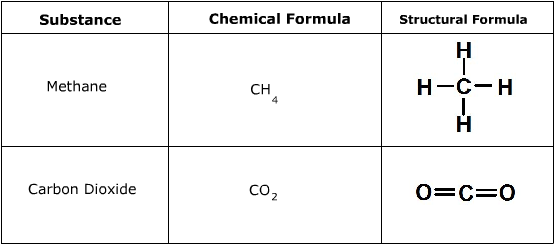# Structural Formula

Structural Formula

A structural formula represents how the atoms in a molecule are arranged in space. It gives an idea of a 3D model of the molecule, which is something that a chemical formula cannot do. The structural formula does not usually indicate the correct bond angles in the molecule.

Structural Formula Examples: